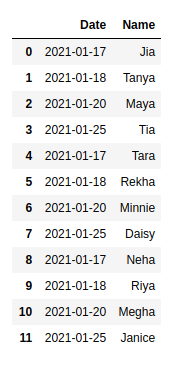Open In App

# How to Merge multiple CSV Files into a single Pandas dataframe ?

While working with CSV files during data analysis, we often have to deal with large datasets. Sometimes, it might be possible that a single CSV file doesn’t consist of all the data that you need. In such cases, there’s a need to merge these files into a single data frame. Luckily, the Pandas library provides us with various methods such as merge, concat, and join to make this possible. Through the examples given below, we will learn how to combine CSV files using Pandas.

File Used:

First CSV –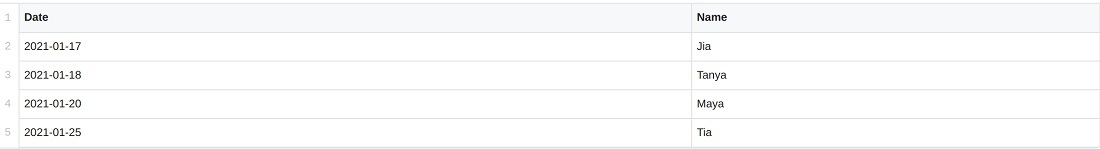Second CSV –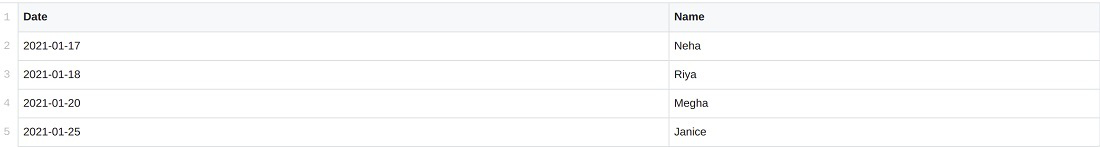Third CSV –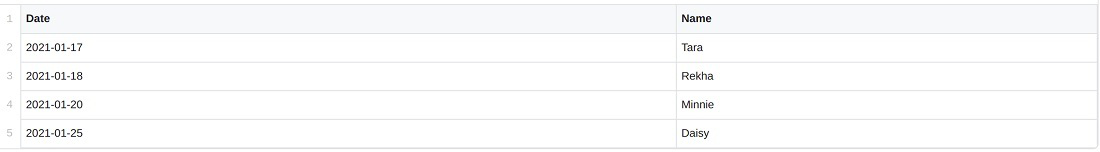### Method 1: Merging by Names

Let us first understand each method used in the program given above:

• pd.concat(): This method stitches the provided datasets either along the row or column axis. It takes the dataframe objects as parameters. Along with that, it can also take other parameters such as axis, ignore_index, etc.
• map(function, iterable): It executes a specified function for each item in iterables. In the example above, the pd.read_csv() function is applied to all the CSV files in the list given.

Approach:

• At first, we import Pandas.
• Using pd.read_csv() (the function), the map function reads all the CSV files (the iterables)  that we have passed. Now, pd.concat() takes these mapped CSV files as an argument and stitches them together along the row axis (default).  We can pass axis=1 if we wish to merge them horizontally along the column. Further,  ignore_index = True sets continuous index values for the merged dataframe.
• The images are given below show mydata.csv, mydata1.csv, and the merged dataframe.

Example:

## Python3

 `# importing pandas``import` `pandas as pd`` ` `# merging two csv files``df ``=` `pd.concat(``    ``map``(pd.read_csv, [``'mydata.csv'``, ``'mydata1.csv'``]), ignore_index``=``True``)``print``(df)`

Output: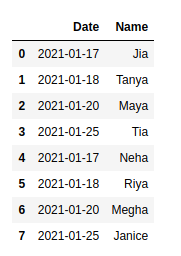### Method 2: Merging All

Approach:

• os.path.join() takes the file path as the first parameter and the path components to be joined as the second parameter. “mydata*.csv helps to return every file in the home directory that starts with “mydata” and ends with .CSV (Use of wildcard *).
• glob.glob() takes these joined file names and returns a list of all these files. In this example, mydata.csv, mydata1.csv, and mydata2.csv are returned.
• Now, just like the previous example, this list of files is mapped and then concatenated.

We can simply write these three lines of code as:

df = pd.concat(map(pd.read_csv, glob.glob(os.path.join(“/home”, “mydata*.csv”))), ignore_index= True)

Example:

## Python3

 `# importing libraries``import` `pandas as pd``import` `glob``import` `os`` ` `# merging the files``joined_files ``=` `os.path.join(``"/home"``, ``"mydata*.csv"``)`` ` `# A list of all joined files is returned``joined_list ``=` `glob.glob(joined_files)`` ` `# Finally, the files are joined``df ``=` `pd.concat(``map``(pd.read_csv, joined_list), ignore_index``=``True``)``print``(df)`

Output: